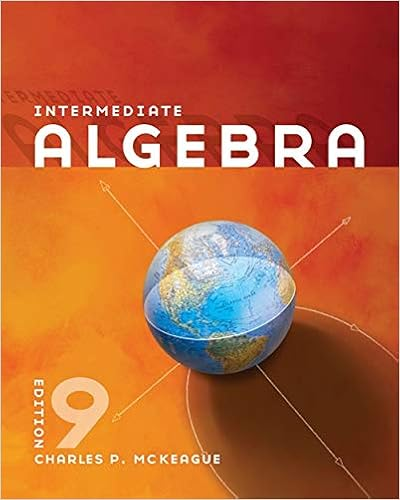# math10bSolFinal - Math 10B Final Exam Solutions 150 pts...

• Notes
• 2

This preview shows page 1 - 2 out of 2 pages.

##### We have textbook solutions for you!
The document you are viewing contains questions related to this textbook.The document you are viewing contains questions related to this textbook.
Chapter 6 / Exercise 17
Intermediate Algebra
MckeagueExpert Verified
Math 10B Final Exam Solutions150 ptsDecember 8, 2009Professor EvansDirections:Answers alone are not sufficient.Justify, and show all work.The multiple partproblems 4,6,7 are worth 18 points each. The other six problems are worth 16 points each.(1)A man was murdered in a 34.6 degree Fahrenheit meat locker. At noon, the corpse temper-ature was 38.6, and an hour later, it had dropped to 36.6. The man was murdered at(a) 6 am(b) 7 am(c) 8 am(d) 9 am(e) none of these timesSolution: LetT(t) denote the temperature of the corpse at timet, with noon being chosenas the “zero hour”t= 0.The solution to the differential equationdT/dt=k(34.6-T) isT(t) = 34.6 +Be-kt. Plug int= 0 to get 38.6 = 34.6 +B, soB= 4 andT= 34.6 + 4e-kt. Plugint= 1 to get 36.6 = 34.6 + 4e-k. Thuse-k= 1/2 andT= 34.6 + 4(1/2)t. At the time of themurder, 98.6 = 34.6 + 4(1/2)t, so 16 = (1/2)t. Thust=-4, so the murder took place at 8 am.
##### We have textbook solutions for you!
The document you are viewing contains questions related to this textbook.The document you are viewing contains questions related to this textbook.
Chapter 6 / Exercise 17
Intermediate Algebra
MckeagueExpert Verified
•••